# ISEE Lower Level Quantitative : How to find a ratio

## Example Questions

2 Next →

### Example Question #581 : Isee Lower Level (Grades 5 6) Quantitative Reasoning

In a litter ofpuppies, there areblack puppies andwhite puppies. What is the ratio of the number of white puppies to black puppies?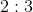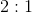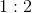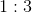Explanation:

The ratio wants us to compare the number of white puppies to the number of black puppies.

We can say the ratio of white puppies to black puppies is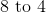. When we write that as a fraction, it becomes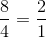, so we can also say that the ratio of white puppies to black puppies is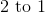or.

### Example Question #582 : Isee Lower Level (Grades 5 6) Quantitative Reasoning

Stacy has started collecting coins.  She has twice as many wheat pennies as she does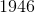dimes, and three times as many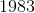quarters as she doesdimes.  If Stacy has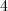dimes, what is the ratio of wheat pennies toquarters?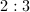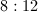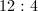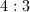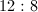Explanation:

We know that Stacy has1946 dimes.

We also know that she has twice as many wheat pennies, so we must multiplyby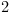to find the total number of wheat pennies.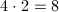We also know that Stacy has three times as many 1986 quarters, so we multiplyby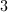to find the total number of 1986 quarters.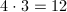Now our ratio of wheat pennies to 1986 quarters is, but this can be simplified because both numbers are divisible by.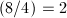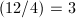So our ratio becomes2 Next →